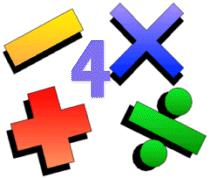Archives

Contribute

 For The Mathematically Inclined

Anil Saigal
09/26/2008

FOR STUDENTS

PROBLEM # 1
In quadrilateral ABCD, angle B is a right angle, diagonal AC is perpendicular to CD. Given: AB=18, BC=21 and CD=14.
Find the perimeter of ABCD.

FOR GROWNUPS

PROBLEM #2
An object moving along a curve in the xy-plane has position (x(t), y(t)) at time t with dx/dt = cos(t^3) and dy/dt = 3 sin(t^2) for 0<=t<=3. At t=2, the object is at position (4,5). Find the total distance traveled by the object over the time period 0<=t<=1.

----------------------------------------------------------------------------------------------------------

Use Problem Solutions M-1002808 as the subject line. Please include your full name in the text of the main message. Everyone with the right answer will be acknowledged in the next issue of Lokvani.

Please do not post your solution in Post Comments. No credit will be given for solutions not sent to anil@lokvani.com.

-------------------------------------------------------------------------------------------------------------

Problem Solutions 09/18/08

PROBLEM # 1
The hypotenuse of a right triangle has length 20 cm. The sum of the lengths of the other two sides is 28 cm. Find the lengths of the other two sides of the triangle.

Solution:
10 cm and 18 cm.

FOR
GROWNUPS
Find an equation of the tangent line to the curve x/y + ( y/x)3 = 2 at the point (– 1, – 1).

Solution:
du/dx = 1.

Congratulations to Aseem Chandawarkar, Maithri Harve, Anupa Manjunatha and Swati Ramanujan who were winners of the last set of puzzles.

|
 1.Здравствуйте March 16, 2020 Podokolzin754 2.приветствую January 31, 2020 Brazhnikov910 3.здравствуйте January 30, 2020 Lupkin797